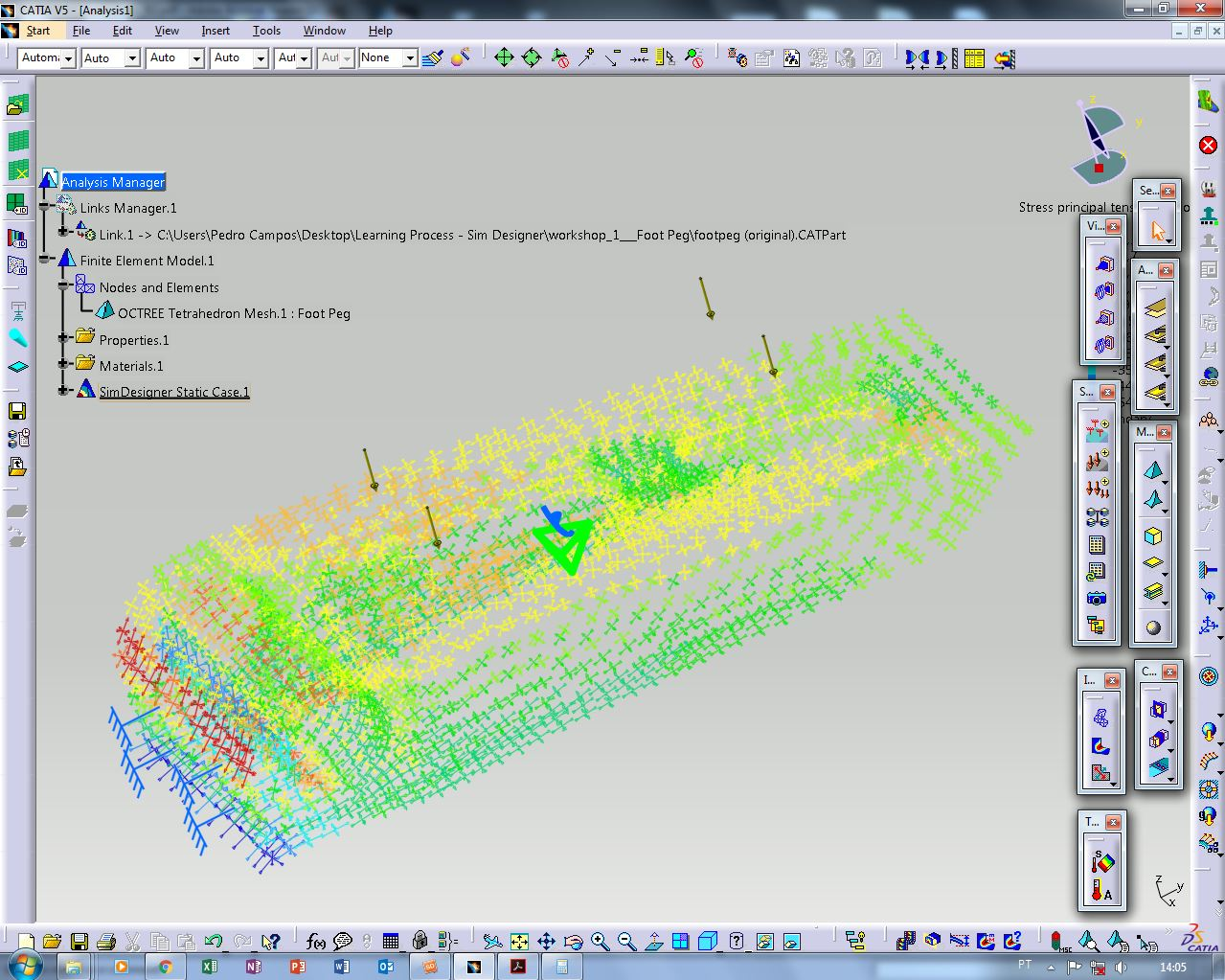# How to do a Basic Linear Finite Element Analysis in CATIA V5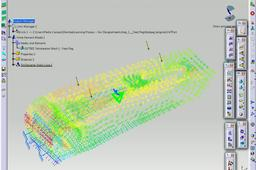After this tutorial, hopefully you will be able to do a simple linear analysis using FEM (Finite Element Method).
The steps are straight forward and the part used is available in my profile (foot peg - ATV).
The idea behind this is seeing how it reacts to a Pressure of 125 KPa.
All the units in this tutorials are in the IS (International System) but i will put the conversions Metric-Imperial.
IF YOU HAVE ANY DOUBTS SUCH AS WHERE ARE THE COMMANDS LOCATED DON'T HESITATE TO CONTACT ME.

Pedro José Silva Campos

1. ### Step 1:

1. OPEN FILE

First, open the file on my profile called "foot peg - ATV".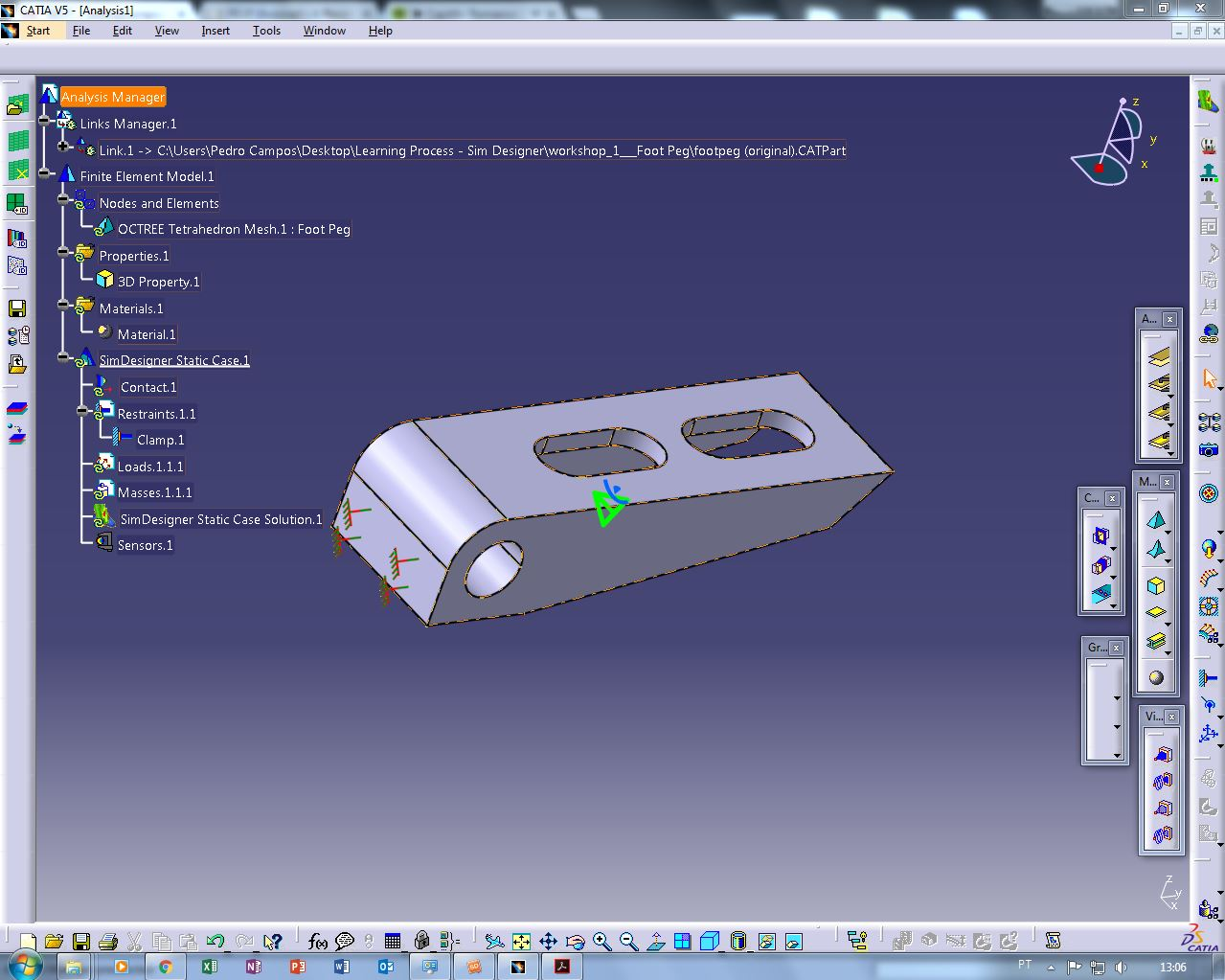2. ### Step 2:

2. SET UNITS and ADD MATERIAL

Set the units either to Metric or Imperial.
Set the material to Aluminium.

3. ### Step 3:

3. START LINEAR WORKBENCH

Start a Linear simulation on the top left.

4. ### Step 4:

4. APPLY CONSTRAINT AND LOAD CONDITIONS

In this case we will use a clamp (full constraint) and apply a pressure of 40 psi or around 175 KPa.
You do this by selecting distributed load and restraint commands on the right (separably) and then the faces you want them applied to.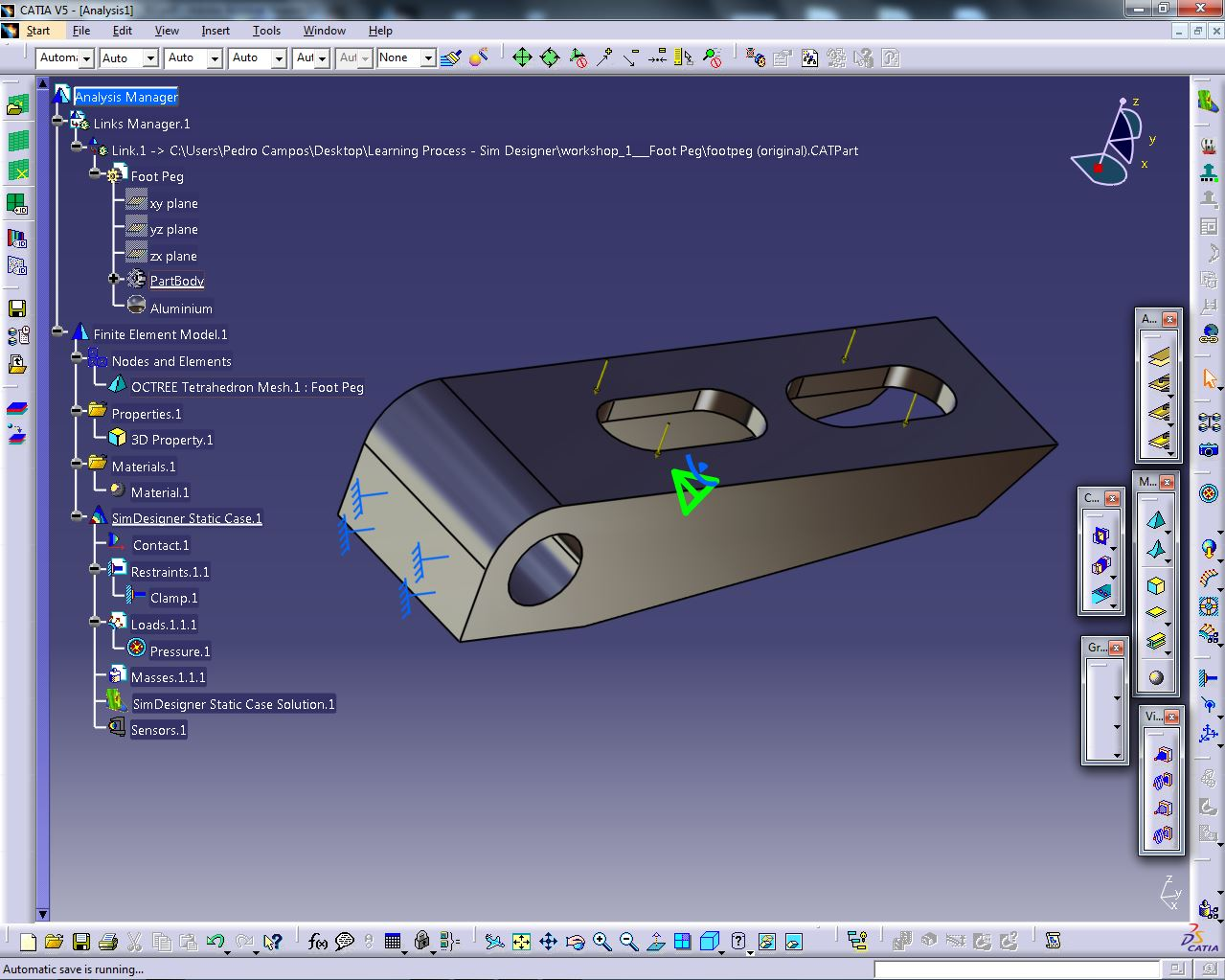5. ### Step 5:

5. SET MESH SIZE

Select Nodes and octree mesh. Press twice and then set size and absolute sag.
In this exaple let's only use linear type elements.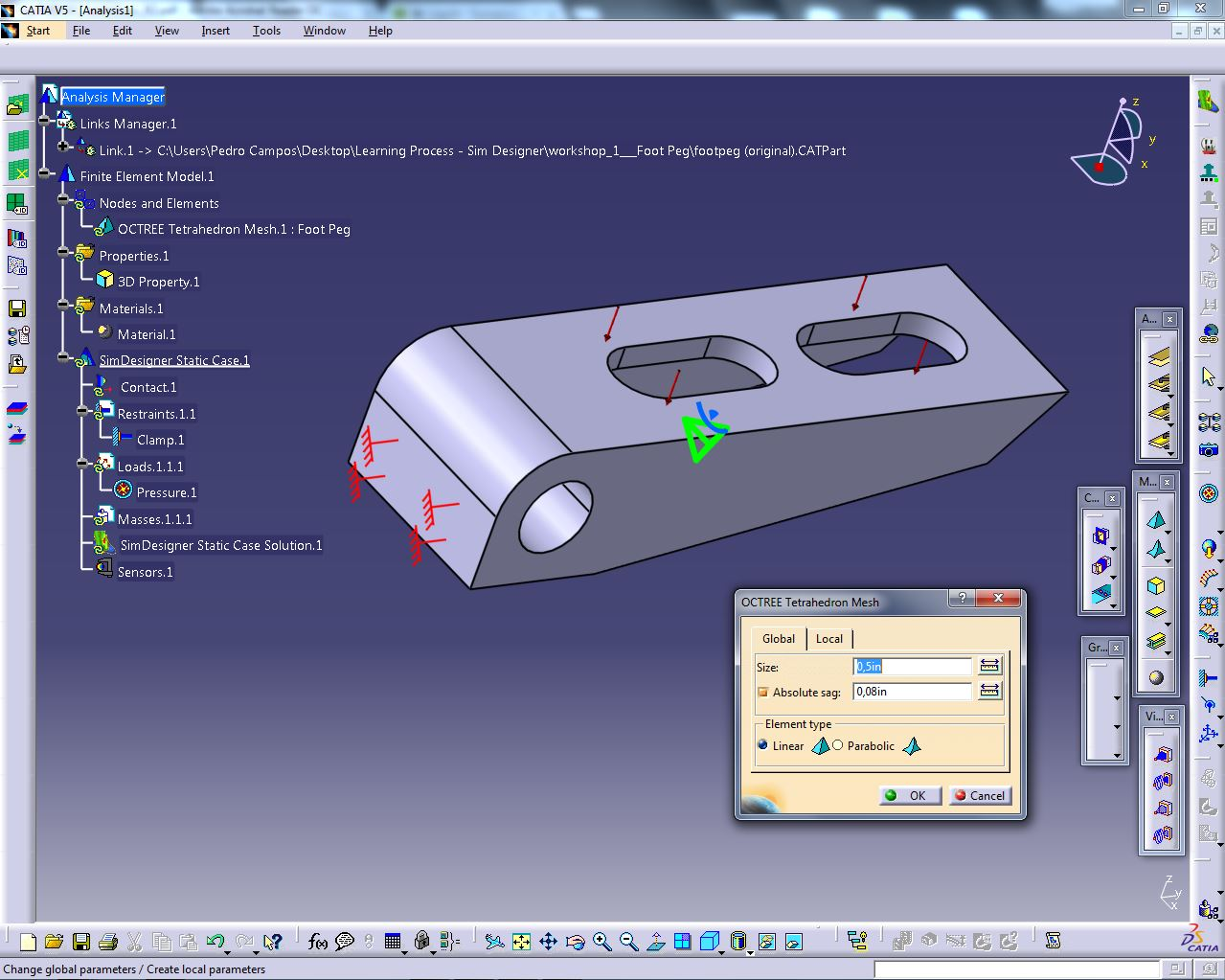6. ### Step 6:

6. RUN THE ANALYSIS / COMPUTE MESH

Press Static Case on the tree and let the analysis run.
Then click close.
The analysis is now complete.
All that is left is to visualize the results.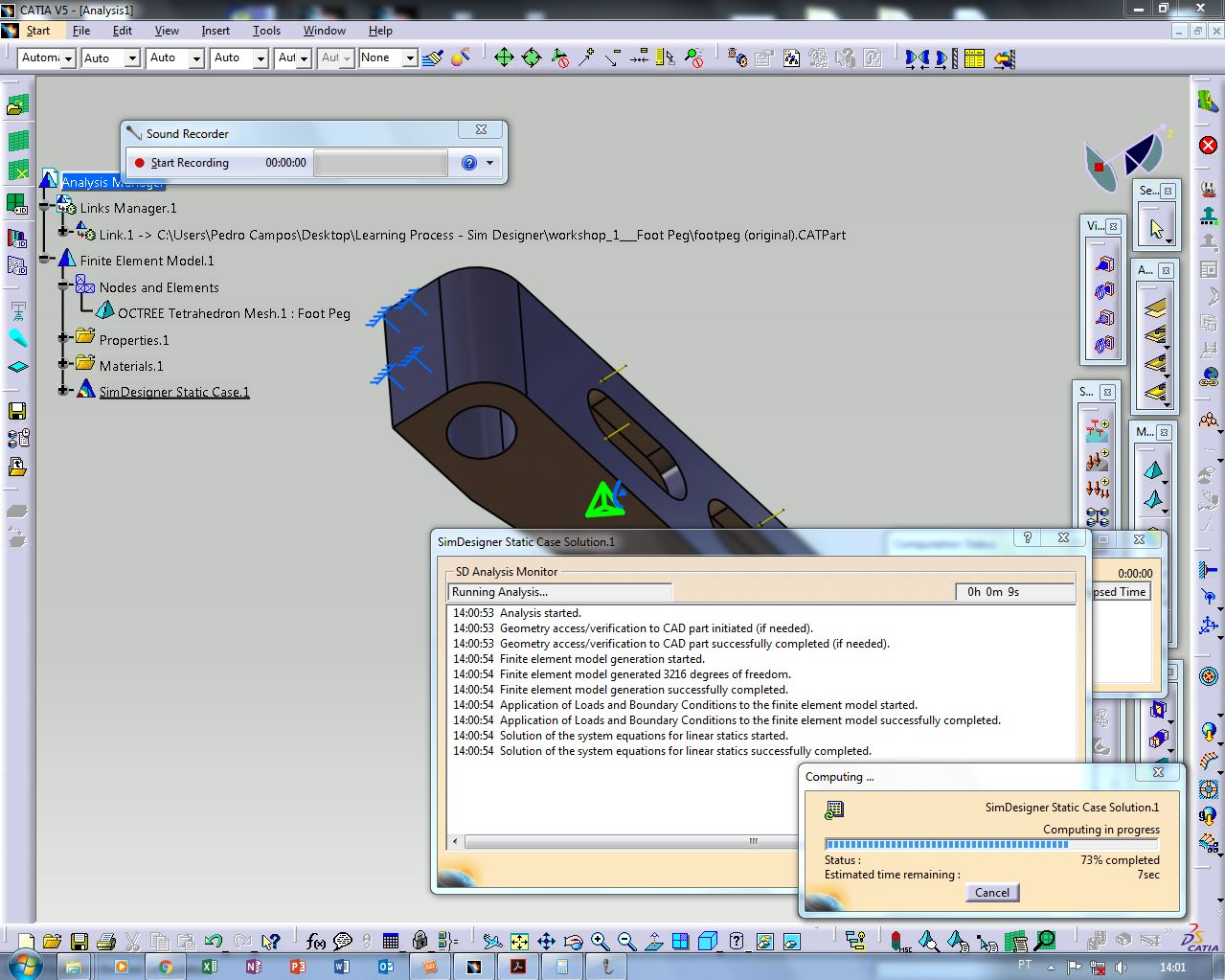7. ### Step 7:

7. VISUALIZING THE RESULTS

View the mesh look and then the different options of visualization.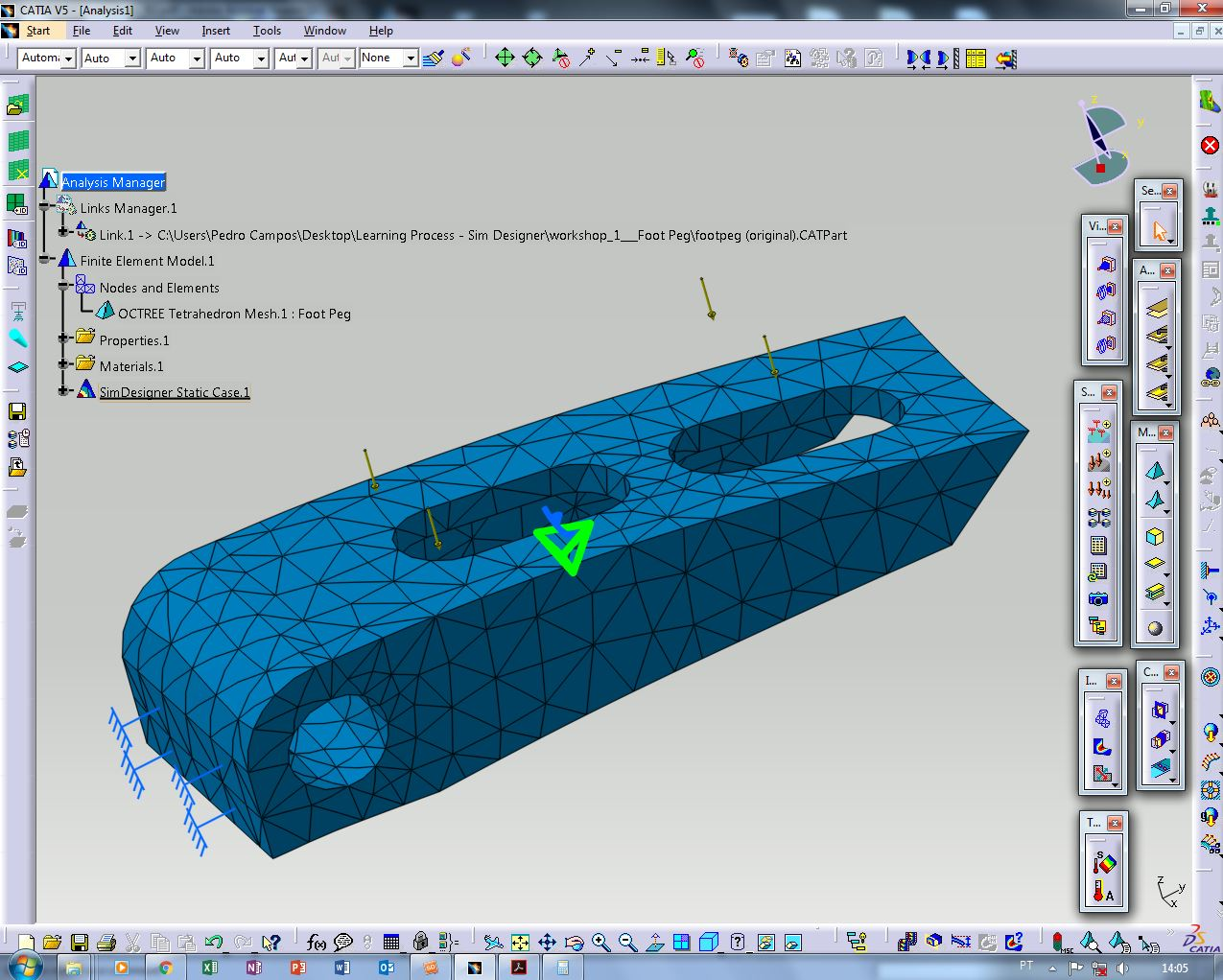8. ### Step 8:

8. RESULT 1: SEE DISPLACEMENT IMAGE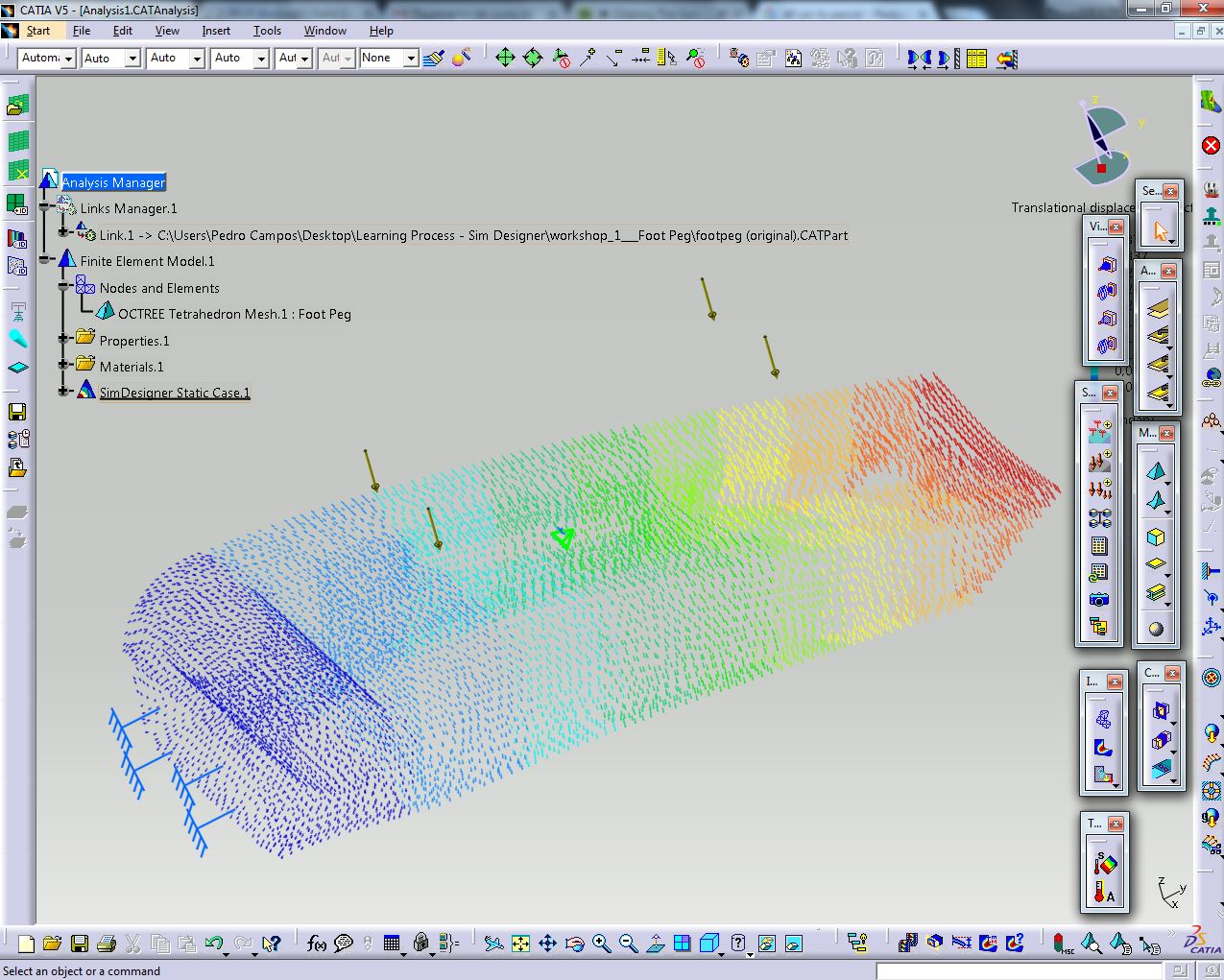9. ### Step 9:

9. RESULT 2: SEE VON MISSES STRESS IMAGE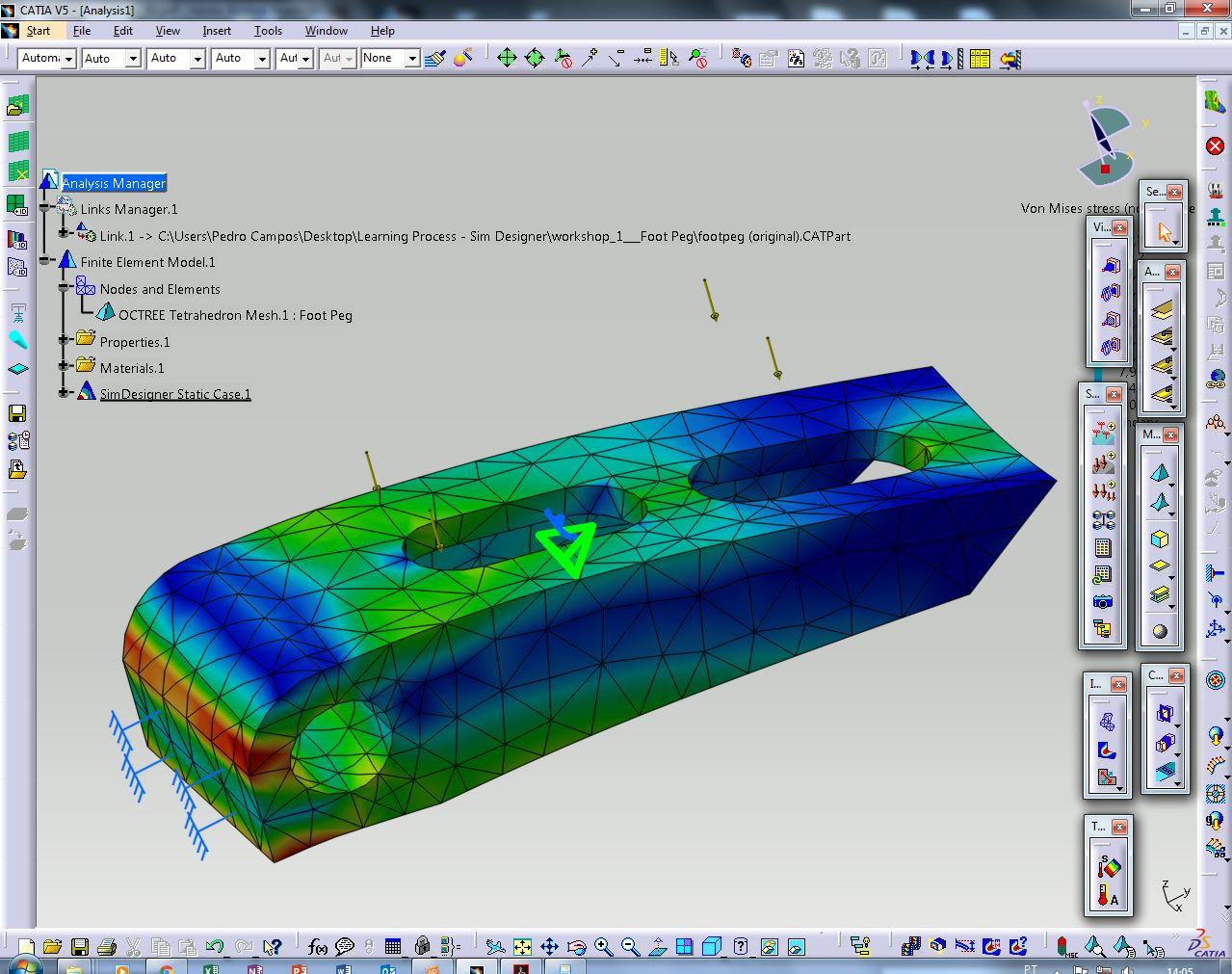10. ### Step 10:

10. RSULT 3: STRESS TENSOR PRINCIPAL